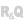HelpWLanguageWLanguage functionsControls, pages and windowsDiagram editor functionsTypes of variablesdiagLibraryPresentationExampleProperties specific to diagLibrary variablesRelated examplesSee alsoDiagramdiagActionAlloweddiagBorderdiagConnectionPointdiagConnectordiagGroupdiagHeaddiagImagediagLayoutdiagLibrarydiagOvaldiagPathdiagPointdiagPolygondiagRectanglediagSelectiondiagShapediagTextdiagWaypoint
• Properties specific to diagLibrary variables
WINDEVWEBDEVWINDEV MobileOthersdiagLibrary (Type of variable)
In french: diagBibliothèque
The diagLibrary type is used to define all the advanced characteristics of a library of preset shapes used by a Diagram Editor control. The characteristics of this library can be defined and changed using different WLanguage properties.
Remark: For more details on the declaration of this type of variable and the use of WLanguage properties, see Declaring a variable.
Example
sFile is string = fTempDir() + [ fSep ] + "custom_library.wddiag"

// Creates a library entirely through programming
TempLibrary is Diagram

D1 is diagOval
D1.Width = 50
D1.Height = 50
D1.Background.Color = DarkRed

D2 is diagOval
D2.Width = 50
D2.Height = 70
D2.Background.Color = DarkGreen

// Use the shapes of the temporary diagram to create the library
// Saves the diagram to the disk
DiagramSave(TempLibrary, sFile)

// Load the diagram as a library
MyLibrary is diagLibrary
MyLibrary.Name = "Custom"

// Adds the library to the Diagram Editor control

ToastDisplay("The custom library has been added to the list.")
MyDiagram is Diagram
// Populate a Looper control with all the shapes from all the libraries
FOR EACH Lib OF MyDiagram.Library
FOR EACH Shape OF Lib
imgShape is Image = DiagramShapeToImage(Shape)
END
END
Properties

Properties specific to diagLibrary variables

The following properties can be used to handle diagLibrary variables:
Property name Type used Effect Name Character string Name of the shape library. Shape Array of diagShape Array of preset shapes.
Remarks
• By default, shape libraries are displayed in the left panel of the Diagram Editor control.
• The Library property of the Diagram Editor control corresponds to an array of variables of type diagLibrary.
Related Examples:Unit examples (WINDEV): The Diagram control [ + ] Simplified use of a Diagram control
Minimum version required
• Version 27